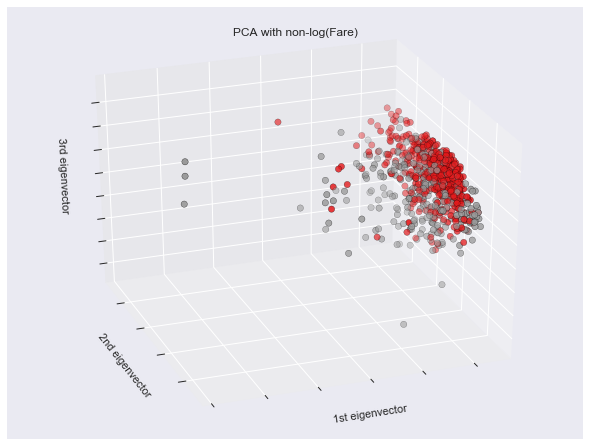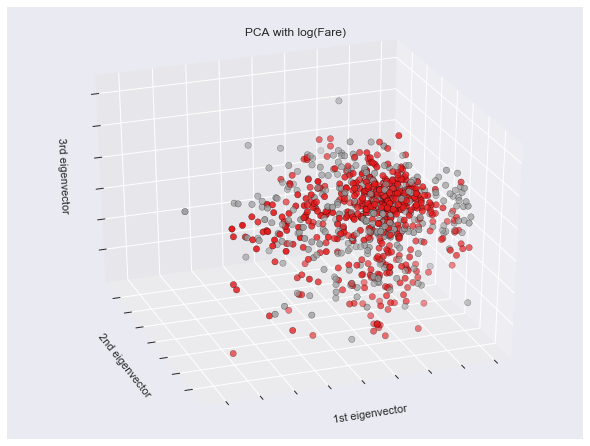#2018 iT 邦幫忙鐵人賽DAY 21
0
AI & Machine Learning

# 資料清理的準備

``````#df指pandas套件中的DataFrame，用來處理表格資料很方便，可說是python中的excel
def preprocess(df):
...
...
return df
``````

# 資料清理(Cleaning)

## 處理遺漏值(Missing Value)

1. 忽略: 這裡必須注意的是，如果你直接把具有遺漏值的資料丟掉，那麼很可能你的訓練資料量或是維度會下降。此外，你在未來預測資料時，也可能會遇到資料不全的狀況，此時總不能夠拒絕預測吧，因次建議的處理方式還是填上一些值，去測試哪一種狀況的訓練結果會比較好。
2. 補上中位數: 比竟平常的狀況會建議補上中位數，這樣相對來說不會受到極端值的影響。
``````df['Fare'] = df['Fare'].fillna(train['Fare'].median())
#直接把中位數填入票價的欄位中
``````
1. 根據原本的資料分布補上亂數
``````# Create a New feature CategoricalAge
age_avg = df['Age'].mean()  #算出Age這個欄位的平均值
age_std = df['Age'].std()  #算出Age這個欄位的標準差
age_null_count = df['Age'].isnull().sum()
#算出有幾個null值。df['Age'].isnull()會讓整個list為null值的部分回變成True(也就是1)，其他變為False(也就是0)。此時，算整個list的總和，就可以算出有多少個null了。
age_null_random_list = np.random.randint(age_avg - age_std, age_avg + age_std, size=age_null_count)
# 產生出一個隨機的list，np.random.randint(最小值, 最大值, size=亂數的長度)。
df['Age'][np.isnan(dataset['Age'])] = age_null_random_list
# df後面第一個中括號索引是column index，後面的中括號則是row index，並將這些欄位填入剛產生好的亂數。
``````
1. 根據可能的狀況補上值: 基本上這個部分要對後面要說的預測方法有點概念，不過基本上就是把遺漏值的欄位，用機器學習的方法或迴歸分析，透過其他欄位進行預測並直接填入預測值。

## 去除極端值(outlier)或是雜訊(noisy data)

1. 偵測: 這個部分可以透過機器學習的分群技術去尋找。也可以透過看到regression，及計算出每個資料點的residual(也就是實際值檢調預測值，也常被代稱為y-yhat)，一旦residual過大，這筆資料就很有可能有某個Feature含有極端值。另外也可以透過PCA降維(decomposition)之後直接查看，很明顯的你會發現有一些點特別跑再外面，此時可能就會存在極端值。(這段code不是出現在上面的function之內的)
``````X = np.matrix(df.drop(['Survived','PassengerId'], axis=1))
Y = np.array(df['Survived'])

fig = plt.figure(1, figsize=(8, 6))
ax = Axes3D(fig, elev=-150, azim=110)

X_reduced = PCA(n_components=3).fit_transform(X)
ax.scatter(X_reduced[:, 0], X_reduced[:, 1], X_reduced[:, 2], c=Y,
cmap=plt.cm.Set1, edgecolor='k', s=40)
ax.set_title("PCA with non-log(Fare)")
ax.set_xlabel("1st eigenvector")
ax.w_xaxis.set_ticklabels([])
ax.set_ylabel("2nd eigenvector")
ax.w_yaxis.set_ticklabels([])
ax.set_zlabel("3rd eigenvector")
ax.w_zaxis.set_ticklabels([])

plt.show()
``````1. 抓錯: 至於要如何判斷錯誤，大多時候還是要依些領域相關的知識的(domain knowledge)。舉例而言，假設今天你有一個大學生的資料集，其中你發現某個現在還在校的大學生，出生日期是1894年，那麼你可能就比需要處理一下這個值。不過這個例子中的知識是我們一般生活中可以判斷出來的知識，假設你今天拿到的是一個很專業的花學相關的資料集，你可能就很難了解，溫度的高低對於化學產品年濁度的影響，甚至相關的單位都沒有概念的時候，可能就比較辛苦了。

## 資料正規化(normalize)

``````def applyfun(row):
row['Fare_log10'] = math.log(row['Fare'], 10) if  row['Fare'] != 0 else 0
return row
df = df.apply(applyfun, axis=1)
``````1. 1000000 == 10^6 => log => 6
2. 10 => 1
3. 1 => 0
4. 0 => inf(無限大)
5. 0.1 => -1
6. 10 ^ -10 => -10

# 資料整合(Integration)

``````def applyfun(row):
row['Family_Size'] = row['SibSp'] + row['Parch']  ## 家庭成員數
row['Is_Mother']= 1 if row['Parch'] > 1 and row['Age']>20 else 0  ## 是否為母親
return row
df = df.apply(applyfun, axis=1)
``````